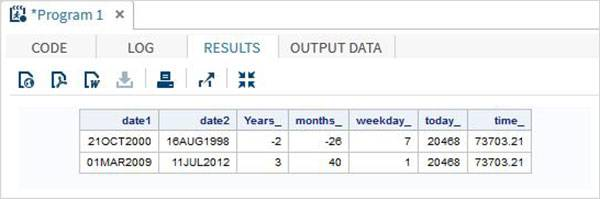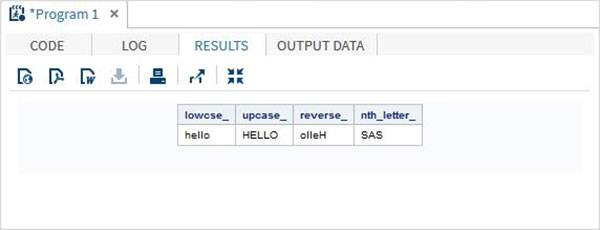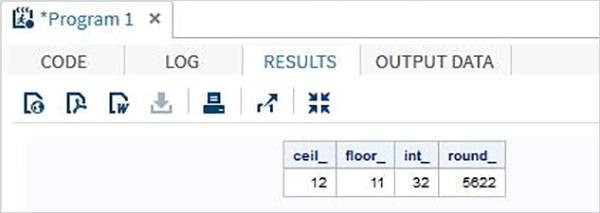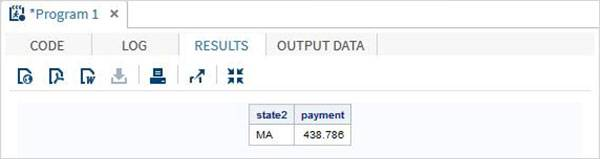# SAS功能

SAS具有多种内置函数，有助于分析和处理数据。 这些函数用作DATA语句的一部分。 它们将数据变量作为参数，并将结果存储到另一个变量中。 根据函数的类型，所需的参数数量可能会有所不同。 一些函数接受零参数，而另一些函数接受固定数量的变量。 以下是SAS提供的功能类型列表。

### 句法

``````FUNCTIONNAME(argument1, argument2...argumentn)
``````

• 数学
• 日期和时间
• 字符
• 舍去

## 数学函数

### 例子

```data Math_functions;
v1=21; v2=42; v3=13; v4=10; v5=29;

/* Get Maximum value */
max_val = MAX(v1,v2,v3,v4,v5);

/* Get Minimum value */
min_val = MIN (v1,v2,v3,v4,v5);

/* Get Median value */
med_val = MEDIAN (v1,v2,v3,v4,v5);

/* Get a random number */
rand_val = RANUNI(0);

/* Get Square root of sum of the values */
SR_val= SQRT(sum(v1,v2,v3,v4,v5));

proc print data = Math_functions noobs;
run;
```

## 日期和时间函数

### 例子

```data date_functions;
INPUT @1 date1 date9. @11 date2 date9.;
format date1 date9.  date2 date9.;

/* Get the interval between the dates in years*/
Years_ = INTCK('YEAR',date1,date2);

/* Get the interval between the dates in months*/
months_ = INTCK('MONTH',date1,date2);

/* Get the week day from the date*/
weekday_ =  WEEKDAY(date1);

/* Get Today's date in SAS date format */
today_ = TODAY();

/* Get current time in SAS time format */
time_ = time();
DATALINES;
21OCT2000 16AUG1998
01MAR2009 11JUL2012
;
proc print data = date_functions noobs;
run;
```## 字符函数

### 例子

```data character_functions;

/* Convert the string into lower case */
lowcse_ = LOWCASE('HELLO');

/* Convert the string into upper case */
upcase_ = UPCASE('hello');

/* Reverse the string */
reverse_ = REVERSE('Hello');

/* Return the nth word */
nth_letter_ = SCAN('Learn SAS Now',2);
run;

proc print data = character_functions noobs;
run;
```## 截断功能

### 例子

```data trunc_functions;

/* Nearest greatest integer */
ceil_ = CEIL(11.85);

/* Nearest greatest integer */
floor_ = FLOOR(11.85);

/* Integer portion of a number */
int_ = INT(32.41);

/* Round off to nearest value */
round_ = ROUND(5621.78);
run;

proc print data = trunc_functions noobs;
run;
```## 其它功能

### 例子

```data misc_functions;

/* Nearest greatest integer */
state2=zipstate('01040');

/* Amortization calculation */
payment=mort(50000, . , .10/12,30*12);

proc print data = misc_functions noobs;
run;
```## 您可能还喜欢：